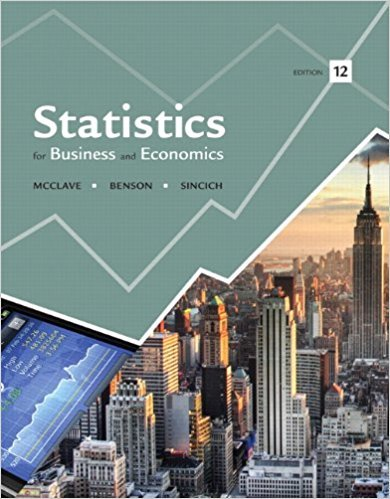×
Get Full Access to Statistics For Business And Economics - 12 Edition - Chapter 9 - Problem 28e
Get Full Access to Statistics For Business And Economics - 12 Edition - Chapter 9 - Problem 28e

×

# Study of recall of TV commercials. Do TV shows withISBN: 9780321826237 51

## Solution for problem 28E Chapter 9

Statistics for Business and Economics | 12th Edition

• Textbook Solutions
• 2901 Step-by-step solutions solved by professors and subject experts
• Get 24/7 help from StudySoup virtual teaching assistantsStatistics for Business and Economics | 12th Edition

4 5 1 239 Reviews
18
4
Problem 28E

Problem 28E

Study of recall of TV commercials. Do TV shows with violence and sex impair memory for commercials? To answer this question, lowa State researchers conducted a designed experiment in which 324 adults were randomly assigned to one of three viewer groups of 108 participants each (Journal of Applied Psychology, June 2002). One group watched a TV program with a violent content code (V) rating, the second group viewed a show with a sex content code (S) rating, and the last group watched a neutral TV program with neither a V nor an S rating. Nine commercials were embedded into each TV show. After viewing the program, each participant was scored on his or her recall of the brand names in the commercial messages, with scores ranging from 0 (no brands recalled) to 9 (all brands recalled). The data (simulated from information provided in the article) are saved in the accompanying file. The researchers compared the mean recall scores of the three viewing groups with an analysis of variance for a completely randomized design.

a. Identify the experimental units in the study.

b. Identify the dependent (response) variable in the study.

c. Identify the factor and treatments in the study.

d. The sample mean recall scores for the three groups were= 2.08,= 1.71, and= 3.17. Explain why one should not draw an inference about differences in the population mean recall scores on the basis of only these summary statistics.

e. An ANOVA on the data yielded the results shown in the Minitab printout on the next page. Locate the test statistic and p-value on the printout.

f. Interpret the results from part

e. using a = 0.01. What can the researchers conclude about the three groups of TV ad viewers?

g. Check that the ANOVA assumptions are reasonably satisfied.Step-by-Step Solution:
Step 1 of 3

Ch12 ­ 10b: Excess returns: MKT 3%, –38% XYZ 7%, –46% Beta = (7 – (–46))/(3 – (–38)) = 1.29 Ch12 ­ 14a: The highest expected return would be if the bond is risk free: y = 2.05% Ch12 ­ 25 a. FCF = (30 – 0.8(30))(1 – 0.40) = 3.6 million Ru = 4% + 1.25 × 5% = 10.25% (the 1.25 is the asset beta (or the unlevered equity beta)) Value today = 3.6/0.1025 = 35.12 million b. FCF without energy = (30 – 18)(1 – 0.40) = 7.2 Cost of capital = 10.25% Energy cost after tax = 3(1 – 0.40) = 1.8 Cost of capital = 4% (because the contract is fixed, no risk) V = 7.2/.1025 – 1.8/0.04 = 70.24 – 45 = 25.24 million c. FCF = 7.2 – 1.8 = 5.4 5.4/25.24 = 21.4% Risk is increased because now energy costs are fixed. Thus a higher cost of capital is appropriate. Ch13 ­ 13 a. \$55. b. Buy if the price goes

Step 2 of 3

Step 3 of 3